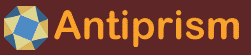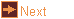# tetra59 - 59 tetrahedra with rational dihedral angles

 Usage    |    Examples    |    Notes

## Usage

```

Usage: tetra59 [options] tetrahedron

Generate 59 Tetrahedra with Rational Dihedral Angles in off format. The 59
Sporadic Tetrahedra is from a paper by Kiran S. Kedlaya, Alexander Kolpakov,
Bjorn Poonen, and Mechael Rubinstein: Space Vectors Forming Rational Angles
The paper can be found at: https://arxiv.org/abs/2011.14232
There are also two special infinite cases included in the findings
The first case was published by M.J.M Hill in 1895. The second case is new.

Options
-h,--help this help message (run 'off_util -H help' for general help)
--version version information
-H        abstract from the paper and description of regge symmetry
-v        verbose output of edge math
-b        verbose output of face math
-l <int>  display the list of Sporadic Tetrahedra as integer=1, degrees=2
-o <file> write output to file (default: write to standard output)

Scene Options
-d <mthd> order of dihedral angles, for matching dihedral angle at position
term 1 (a12) for regge symmetry (also permutes special cases)
angles permuted as pairs: 1-(a12,a34) 2-(a13,a24) 3-(a14,a23)
for each pair in position one, there are two arrangements
a:1,2,3  b:1,3,2; c:2,1,3  d:2,3,1; e:3,1,2  f:3,2,1 (default:a)
-p <int>  build regge pair if found (1 or 2) not for special cases (sets -z)
-r        reflect
-z        scale volume to 1

Special Cases
-s <int>  special case 1 or 2 (default: none)
case 1: (pi/2, pi/2, pi - 2x, pi/3, x, x)
for pi/6 < x < pi/2 (30 < x < 90 degrees)
case 2: (5pi/6 - x, pi/6 + x, 2pi/3 - x, 2pi/3 - x, x, x)
for pi/6 < x <= pi/3 (30 < x <= 60 degrees)
-a <ang>  angle in degrees (default: 45)
-w        allow any angle for case s (for testing case 2, 60 < x < 90)

Coloring Options (run 'off_util -H color' for help on color formats)
-V <col>  vertex color (default: gold)
-E <col>  edge color   (default: lightgray)
-f <mthd> mthd is face coloring method using color in map (default: u)
keyword: none - sets no color
u - unique coloring
s - symmetric coloring
r - regge group (1-16) (not for special cases)
-T <tran> face transparency. valid range from 0 (invisible) to 255 (opaque)
-m <maps> color maps for faces to be tried in turn
(default for -f r: rainbow16, otherwise compound)

```

## Examples

list of 59 tetrahedra with rational dihedral angles in integer factors
```tetra59 -l int
```

list of 59 tetrahedra with rational dihedral angles in degrees
```tetra59 -l deg
```

display a tetrahedra
```tetra59 12 | antiview -v 0.01
```

display a tetrahedra with a unique color given by its regge class
```tetra59 38 -f r | antiview -v 0.01
```

display special case 2 tetrahedron with angle set at 40 degrees
```tetra59 -s 2 -a 40 | antiview -v 0.01
```

compare two tetrahedra of the same volume in the same Regge class
```tetra59 24 -z > tmp.off
tetra59 29 -z | antiview - tmp.off
```

compare two tetrahedra of the same volume in the same Regge class use the -p option to find the match of the dihedral pair (6, 6) in the second column set by -d c. It finds the matching pair in tetrahedron 14 in the first column. Use tetra59 -l deg to list standardized angles for easier matching
```tetra59 13 -d c -p 1 | antiview
```

## Notes

tetra59 was written by Roger Kaufman. Based on a paper by Kiran S. Kedlaya, Alexander Kolpakov, Bjorn Poonen, and Mechael Rubinstein: Space Vectors Forming Rational Angles https://arxiv.org/abs/2011.14232.

The following extended help for the program may be displayed with tetra59 -H

```

The project was undertaken in memory of John H. Conway

Abstract. We classify all sets of nonzero vectors in R3 such that the angle
formed by each pair is a rational multiple of Pi .The special case of
four-element subsets lets us classify all tetrahedra whose dihedral angles are
multiples of Pi, solving a 1976 problem of Conway and Jones: there are 2
one-parameter families and 59 sporadic tetrahedra, all but three of which are
related to either the icosidodecahedron or the B3 root lattice. The proof
requires the solution in roots of unity of a W(D6)-symmetric polynomial
equation with 105 monomials (the previous record was 12 monomials).

A brief description of a Regge Symmetry group, a mathematical symmetry.

For all the tetrahedra in a Regge Symmetry group, if given equal volume they
will have the following characteristics

1) For all the tetrahedrons in the group, the sum of the 6 edges will be equal
2) In the group, for a tetrahedron with edges (x,y,a,b,c,d) there may be one
or more tetrahedrons with edges x,y,s-a,s-b,s-c,s-d where s = (a+b+c+d)/2
The tetrahedra come in pairs by dihedral angle. There may be more than one
pairing depending on the three sets of opposing angles. Of the pairings...
3) The two opposing dihedral angles at edges x and y will be equal
4) The edge lengths of x and y of the two tetrahedra will be equal
5) If e,f,g,h are the dihedral angles at the edges a,b,c,d then the dihedral
angles at edges s-a,s-b,s-c,s-d are t-e,t-f,t-g,t-h where t = (e+f+g+h)/2

Method of Building Tetrahedrons

The tetrahedrons vertices are numbered 1,2,3 and 4. The dihedral angles given
for the six edges from the paper are (a12,a34,a13,a24,a14,a23). An edge length
of 1 is drawn from the origin to x,y,z = 0,1,0. Face angles for face A and B
are calculated from dihedral angles using trigonometry. Edge a (e13) and
edge b (e14) lengths are calculated from face angles from A and B angles using
trigonometry. Edge a (e13) is drawn rotated into place on the xy plane. Edge
b (e14) is also drawn and rotated on the xy plane and additionally rotated on
the Y axis by dihedral angle a12. At this point 4 vertices exist in 3D space
and the four faces A, B, C and D can be drawn.

Comparing Two Tetrahedra in the Same Regge Group

In a Regge group, two tetrahedra that share the same dihedral angle pair can
be compared and Regge symmetry math demonstrated. Not all angle pairs are in
the same columns pairs. The -d option can choose which order to place the
dihedral pairs so that the pairs to be compared will be moved to the first
column. The -p option will find the pairing if it exists and move its order
to the first column. -p can take 1 or 2 depending on which order it picks for
the second and third column. The listing -l deg to list the 59 tetrahedra in
terms of degrees will help see which pairing are valid.

```